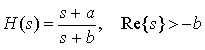## Response of LTI Systems Using Laplace Transforms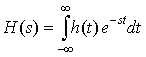where h(t) is an impulse response, is called the system function or transfer function and it completely characterizes the input/output relationship of an LTI system. We can use it to determine time responses of LTI systems.

### Transfer Functions

We can use Laplace Transforms to solve differential equations for systems (assuming the system is initially at rest for one-sided systems) of the form: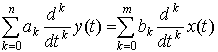Taking the Laplace Transform of both sides of this equation and using the Differentiation Property, we get: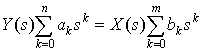From this, we can define the transfer function H(s) as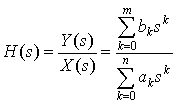### Partial Fraction Expansion

Instead of taking contour integrals to invert Laplace Transforms, we will use Partial Fraction Expansion. We review it here. Given a Laplace Transform,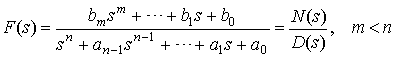We write its Partial Fraction Expansion as: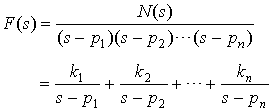where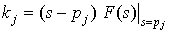is the residue of the pole at pj.

Thus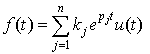because the Inverse Laplace Transform of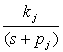is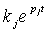Example 1  Find y(t) where the transfer function H(s) and the input x(t) are given. Use Partial Fraction Expansion to find the output y(t):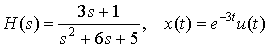Example 2  Find the transfer function H(s) for the differential equation. Assume zero initial conditions.

y'(t) + 2y(t) = 3x'(t).

Example 3  Now let the input to the system be x(t) = 5u(t). Find y(t).

### Convolution

As we saw for the Fourier Transform

x(t)*h(t) ↔ X(s)H(s)

This is useful for studying LTI systems. In fact, we can completely characterize an LTI system from:

1. The system differential equation
2. or the system transfer function H(s)
3. or the system impulse response h(t).

Example 4  Find the step response s(t) to

h(t) = e-tu(t)

 Hint: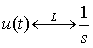Example 5  Find the output of an LTI system with impulse response h(t) = ebtu(t) to an input x(t) = eatu(t), where a ≠ b.

## LTI System Characteristics

### Stability

We saw that a condition for bounded-input bounded-output stability was: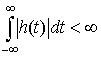Let's look at stability from a system function standpoint. Given a Laplace Transform H(s), we expand H(s) with Partial Fraction Expansion: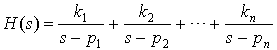The corresponding impulse response is: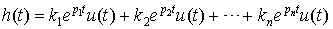What happens to h(t) as t → ∞? For a system to be stable, its impulse response must not blow up as t → ∞.

 If Re{pi},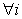, then h(t) decays to 0 as t → ∞ and the system is stable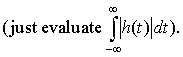Therefore, the system is BIBO stable if and only if all poles of H(s) are in the left half plane of the s-plane.

If you study CONTROL THEORY, you will learn more about this. Using feedback , you can build systems to steer the poles into the left half plane and thus stabilize the system. Here is an example of such a system.

Example 6  FEEDBACK

1. You are given a system with impulse response

h(t) = etu(t)

Is the system stable?

2. You now hook up the system up into a "Feedback" system as shown. Find the new impulse response or transfer function. Find the range on the parameter A such that the system is stable.

### Invertibility

You can find the inverse of a system using Laplace Transforms. This is because: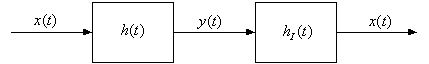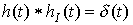Take the Laplace Transform of both sides of this equation: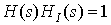Therefore, the Laplace Transform of the inverse system is simply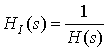Example 7  Find the inverse system of